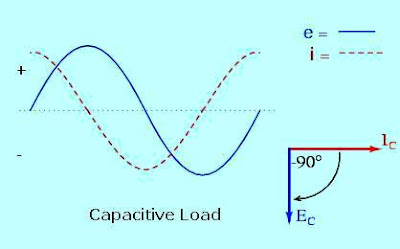### Electrical Load Classification and Types – Part Two

In the previous topic, Electrical Load Classification and Types – Part One, I listed all the Electrical Load Classifications and Types and today I will begin to explain each electrical load type in more detail.

1-

Electrical Loads can be classified according to the nature of the load as follows:

The Resistive Electrical Loads naturally resist the flow of electricity through it by converting some of this electrical energy into heat (thermal energy) , the result will be a drop in the amount of electrical energy transferred through it.

a- Incandescent light bulbs:
An incandescent light bulb produces light by passing an electric current through a filament in a vacuum. The resistance of the filament causes it to heat up and the electrical energy is converted to light energy.

b- Electric heaters:
Electric heaters work in the same way, its resistance convert the electrical energy to thermal energy (heat) and they may produce little, if any, light.

Characteristics of Resistive Electrical Loads:(see fig.1)

• Resistance (R) is measured in ohms.
• The electrical current and the voltage in a resistive load are said to be "in phase" with each other. As voltage rises or falls, the current also rises and falls with it.
• As the voltage and current are in phase, the power factor is in unity.
• Resistive loads not having any significant inrush current. When a resistive load is energized, the current rises instantly to its steady-state value, without first rising to a higher value.fig (1): Characteristics of Resistive Electrical Loads

A capacitor stores electrical energy. Two conductive surfaces are separated by a non-conductive insulator.

When an electrical current is applied to a capacitor, electrons from the current gather on the plate attached to the terminal to which the electric current is applied. When the current is removed, the electrons will to flow back through the circuit to reach the other terminal of the capacitor.

Capacitors are used in electric motors, radio circuits, power supplies and many other circuits.

(see fig.2)

• The capability of a capacitor to store electrical energy is called capacitance (C). The main unit of measure is the farad, but most capacitors are measured in microfarads.
• The current leads the voltage of a capacitor. The voltage across the terminals starts out at zero volts while the current is at its maximum. As the charge builds on the capacitors plate, the voltage rises and the current falls. As a capacitor discharges, the current rises as the voltage falls.
• The current waveform is leading the voltage waveform; therefore, the voltage peaks and current peaks are not in phase. The amount of phase delay is given by the cosine of the angel (Cos) between the vectors representing voltage and current.fig (2): Characteristics of Capacitive Electrical Loads

An inductor may be any conductive material. When a changing current passes through an inductor, it induces a magnetic field around itself. Turning the inductor into a coil increases the magnetic field. A similar principal occurs when a conductor is placed within a changing magnetic field. The magnetic field induces an electrical current within the conductor.

Examples of inductive loads include transformers, electric motors and coils. Two sets of magnetic fields in an electric motor oppose each other, forcing the motor's shaft to spin.

A transformer has two inductors, a primary and a secondary. The magnetic field in the primary winding induces an electric current in the secondary winding.

A coil stores energy in the magnetic field it induces when a changing current passes through it and releases the energy when the current is removed.

(see fig.3)

• Inductance (L) is measured in henries.
• The changing voltage and current in an inductor are out of phase. As current rises to a maximum, the voltage falls.
• The current waveform is lagging behind the voltage waveform, therefore, the voltage peaks and current peaks are not in phase. The amount of phase delay is given by the cosine of the angel (Cos) between the vectors representing voltage and current.
• Inductive load pulls a large amount of current (an inrush current) when first energized. After a few cycles or seconds the current "settles down" to the full-load running current.
• The time required for the current to "settle down" depends on the frequency or/and the inductance value of the Inductive load.fig (3): Characteristics of Inductive Electrical Loads

a- Cable and Conductors
All conductors have some resistance under normal conditions and also exhibit inductive and capacitive influences, but these small influences are generally dismissed for practical purposes.

The tuning circuit of a radio uses variable inductors or capacitors in combination with a resistor to filter out a range of frequencies while allowing just one narrow band to pass through to the rest of the circuit.

c- Cathode Ray Tube
A cathode ray tube in a monitor or television makes use of inductors, resistors and the inherent capacitance of the tube to control and display a picture on the phosphor coatings of the tube.

d- Motors
Single phase motors often use capacitors to aid the motor during starting and running. The start capacitor provides an additional phase of voltage to the motor since it shifts the current and voltage out of phase with each other.

(see fig.4)

The phase angle, φ, may be positive or negative depending on whether the overall voltage (the sum of the voltage phasors) leads or lags the current in the circuit (which is the same everywhere).fig (4): Characteristics of Combination Electrical Loads

In the next topic, I will continue explaining Other Types of Electrical Loads. So, please keep following.

1.Hmm topic is good but I want to know how we can explain the importance od electrical load to others such as mechanical & process engineers. so we can get the same with any argument to start the electrical work

2.Yes, very good information! I understtod very well.

3.Good Job keep it up

4.Good Job keep it up